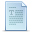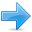# Classes (II)

C++ incorporates the option to use standard operators to perform operations with classes in addition to with fundamental types. For example:

 ``12`` ``````int a, b, c; a = b + c;``````

This is obviously valid code in C++, since the different variables of the addition are all fundamental types. Nevertheless, it is not so obvious that we could perform an operation similar to the following one:

 ``12345`` ``````struct { string product; float price; } a, b, c; a = b + c;``````

In fact, this will cause a compilation error, since we have not defined the behavior our class should have with addition operations. However, thanks to the C++ feature to overload operators, we can design classes able to perform operations using standard operators. Here is a list of all the operators that can be overloaded:

```+    -    *    /    =
>    +=   -=   *=   /=

>>

=  >>=  ==   !=
=   >=   ++   --   %    &    ^    !    |
~    &=   ^=   |=   &&   ||   %=   []   ()   ,    ->*  ->   new
delete    new[]     delete[]
```

To overload an operator in order to use it with classes we declare operator functions, which are regular functions whose names are the operator keyword followed by the operator sign that we want to overload. The format is:

type operator sign (parameters) { /*...*/ }

Here you have an example that overloads the addition operator (+). We are going to create a class to store bidimensional vectors and then we are going to add two of them: a(3,1) and b(1,2). The addition of two bidimensional vectors is an operation as simple as adding the two x coordinates to obtain the resulting x coordinate and adding the two y coordinates to obtain the resulting y. In this case the result will be (3+1,1+2) = (4,3).

 ``````// vectors: overloading operators example #include using namespace std; class CVector { public: int x,y; CVector () {}; CVector (int,int); CVector operator + (CVector); }; CVector::CVector (int a, int b) { x = a; y = b; } CVector CVector::operator+ (CVector param) { CVector temp; temp.x = x + param.x; temp.y = y + param.y; return (temp); } int main () { CVector a (3,1); CVector b (1,2); CVector c; c = a + b; cout << c.x << "," << c.y; return 0; }`````` `4,3`

It may be a little confusing to see so many times the CVector identifier. But, consider that some of them refer to the class name (type) CVector and some others are functions with that name (constructors must have the same name as the class). Do not confuse them:

 ``12`` ``````CVector (int, int); // function name CVector (constructor) CVector operator+ (CVector); // function returns a CVector ``````

The function operator+ of class CVector is the one that is in charge of overloading the addition operator (+). This function can be called either implicitly using the operator, or explicitly using the function name:

 ``12`` ``````c = a + b; c = a.operator+ (b);``````

Both expressions are equivalent.

Notice also that we have included the empty constructor (without parameters) and we have defined it with an empty block:

 ``1`` ``CVector () { };``

This is necessary, since we have explicitly declared another constructor:

 ``1`` ``CVector (int, int);``

And when we explicitly declare any constructor, with any number of parameters, the default constructor with no parameters that the compiler can declare automatically is not declared, so we need to declare it ourselves in order to be able to construct objects of this type without parameters. Otherwise, the declaration:

 ``1`` ``CVector c;``

included in main() would not have been valid.

Anyway, I have to warn you that an empty block is a bad implementation for a constructor, since it does not fulfill the minimum functionality that is generally expected from a constructor, which is the initialization of all the member variables in its class. In our case this constructor leaves the variables x and y undefined. Therefore, a more advisable definition would have been something similar to this:

 ``1`` ``CVector () { x=0; y=0; };``

which in order to simplify and show only the point of the code I have not included in the example.

As well as a class includes a default constructor and a copy constructor even if they are not declared, it also includes a default definition for the assignment operator (=) with the class itself as parameter. The behavior which is defined by default is to copy the whole content of the data members of the object passed as argument (the one at the right side of the sign) to the one at the left side:

 ``123`` ``````CVector d (2,3); CVector e; e = d; // copy assignment operator ``````

The copy assignment operator function is the only operator member function implemented by default. Of course, you can redefine it to any other functionality that you want, like for example, copy only certain class members or perform additional initialization procedures.

The overload of operators does not force its operation to bear a relation to the mathematical or usual meaning of the operator, although it is recommended. For example, the code may not be very intuitive if you use operator + to subtract two classes or operator== to fill with zeros a class, although it is perfectly possible to do so.

Although the prototype of a function operator+ can seem obvious since it takes what is at the right side of the operator as the parameter for the operator member function of the object at its left side, other operators may not be so obvious. Here you have a table with a summary on how the different operator functions have to be declared (replace @ by the operator in each case):

ExpressionOperatorMember functionGlobal function
@a+ - * & ! ~ ++ --A::operator@()operator@(A)
a@++ --A::operator@(int)operator@(A,int)
a@b+ - * / % ^ & | < > == != <= >= << >> && || ,A::operator@ (B)operator@(A,B)
a@b= += -= *= /= %= ^= &= |= <<= >>= []A::operator@ (B)-
a(b, c...)()A::operator() (B, C...)-
a->x->A::operator->()-
Where a is an object of class A, b is an object of class B and c is an object of class C.

You can see in this panel that there are two ways to overload some class operators: as a member function and as a global function. Its use is indistinct, nevertheless I remind you that functions that are not members of a class cannot access the private or protected members of that class unless the global function is its friend (friendship is explained later).

### The keyword this

The keyword this represents a pointer to the object whose member function is being executed. It is a pointer to the object itself.

One of its uses can be to check if a parameter passed to a member function is the object itself. For example,

 ``````// this #include using namespace std; class CDummy { public: int isitme (CDummy& param); }; int CDummy::isitme (CDummy& param) { if (¶m == this) return true; else return false; } int main () { CDummy a; CDummy* b = &a; if ( b->isitme(a) ) cout << "yes, &a is b"; return 0; }`````` `yes, &a is b`

It is also frequently used in operator= member functions that return objects by reference (avoiding the use of temporary objects). Following with the vector's examples seen before we could have written an operator= function similar to this one:

 ``123456`` ``````CVector& CVector::operator= (const CVector& param) { x=param.x; y=param.y; return *this; }``````

In fact this function is very similar to the code that the compiler generates implicitly for this class if we do not include an operator= member function to copy objects of this class.

### Static members

A class can contain static members, either data or functions.

Static data members of a class are also known as "class variables", because there is only one unique value for all the objects of that same class. Their content is not different from one object of this class to another.

For example, it may be used for a variable within a class that can contain a counter with the number of objects of that class that are currently allocated, as in the following example:

 ``````// static members in classes #include using namespace std; class CDummy { public: static int n; CDummy () { n++; }; ~CDummy () { n--; }; }; int CDummy::n=0; int main () { CDummy a; CDummy b; CDummy * c = new CDummy; cout << a.n << endl; delete c; cout << CDummy::n << endl; return 0; }`````` ```7 6```

In fact, static members have the same properties as global variables but they enjoy class scope. For that reason, and to avoid them to be declared several times, we can only include the prototype (its declaration) in the class declaration but not its definition (its initialization). In order to initialize a static data-member we must include a formal definition outside the class, in the global scope, as in the previous example:

 ``1`` ``int CDummy::n=0;``

Because it is a unique variable value for all the objects of the same class, it can be referred to as a member of any object of that class or even directly by the class name (of course this is only valid for static members):

 ``12`` ``````cout << a.n; cout << CDummy::n;``````

These two calls included in the previous example are referring to the same variable: the static variable n within class CDummy shared by all objects of this class.

Once again, I remind you that in fact it is a global variable. The only difference is its name and possible access restrictions outside its class.

Just as we may include static data within a class, we can also include static functions. They represent the same: they are global functions that are called as if they were object members of a given class. They can only refer to static data, in no case to non-static members of the class, as well as they do not allow the use of the keyword this, since it makes reference to an object pointer and these functions in fact are not members of any object but direct members of the class.Previous:Classes (I)IndexNext:Friendship and inheritance